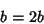## Fallacy

A fallacy is an incorrect result arrived at by apparently correct, though actually specious reasoning. The most common example of a mathematical fallacy is the proof'' thatas follows. Let, thenThe incorrect step is division by(equal to 0), which is invalid. Ball and Coxeter (1987) give other such examples in the areas of both arithmetic and geometry.

References

Ball, W. W. R. and Coxeter, H. S. M. Mathematical Recreations and Essays, 13th ed. New York: Dover, pp. 41-45 and 76-84, 1987.

Pappas, T. Geometric Fallacy & the Fibonacci Sequence.'' The Joy of Mathematics. San Carlos, CA: Wide World Publ./Tetra, p. 191, 1989.